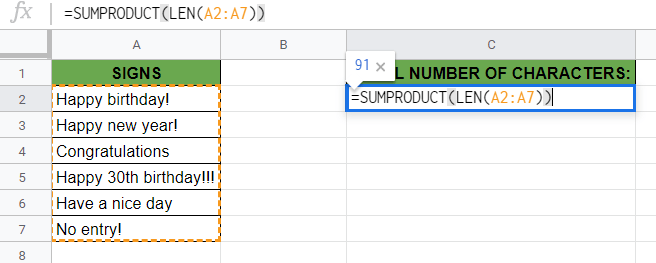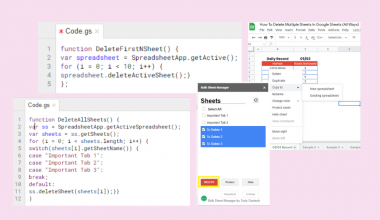# How to Use LEN Function in Google Sheets

The LEN function in Google Sheets is useful to return the length or the number of characters of a string.

The `LEN` function is a really simple text function that we use regularly. It’s used for counting the number of characters in a string (a textual value).

It’s also useful to include in other functions where the length of a specific string is required dynamically.

Let’s take an example.

Say you create wishing signs from a special material and you create the signs letter by letter. 🎉

You need to know the total number of characters you have to prepare to complete all the orders.

So how do we do that?

Simple. The `LEN` function just needs an input value, and it will automatically return the number of characters in this value.

Let’s go straight into real examples where we will deal with actual values to see how we can write our own LEN function in Google Sheets.

## The Anatomy of the LEN Function

The syntax (the way we write) the `LEN` function is especially simple, and it is as follows:

`=LEN(text)`

Let’s break this down to understand the syntax of `LEN` function and each term mean:

• `=` the equal sign is how we begin any function in Google Sheets.
• `LEN` this is our function. We need to add the `text` attribute for it to work properly.
• `text` is the only attribute of this function. It is the input string whose length the formula will return.

Not only the `LEN` function counts the letters but also all characters, even spaces and nonprinting characters. If your `LEN` formula returns unexpected values, then ensure that there are no such characters in the text.

## A Real Example of Using LEN Function

Let’s look at the examples below to see how to use the LEN function in Google Sheets.

### Count Characters in a Cell in Google Sheets

This is the simplest problem. We want to use the `LEN` function to count the number of characters in a cell.As always, we can pass the text value to the function both as a direct value (in cell A2) or as a cell reference (in cell B2). The function accepts direct text values within a pair of double quotes, and a simple cell reference without any quotes as well.

The function with a direct text value is as follows:

`=LEN("Happy Birthday!")`

And the function with a cell reference is:

`=LEN(B3)`

As a result, we get 15. The formula outputs the length of the text attribute (“Happy birthday!“) in both cases. Note that every character, including spaces and the exclamation mark, is included in the length.

It’s easy, right?

### Count Characters in a Range of Cells in Google Sheets

This example below shows precisely how the `LEN` function works to count the number of characters in a range in Google Sheets.

Let’s see the example described above. You create wishing signs, and you need to know the total number of characters that these phrases contain.As you can see, it’s also possible to count the total number of characters in a range of cells. So we summed all the characters that are present in the cells and the function returned one single number (“91“) that is the sum of all the lengths of cells.The function is as follows:

`=SUMPRODUCT(LEN(A2:A7))`

Here’s what this example does:

• We selected a random cell where we wanted to show the result and started off writing a formula with an ‘=’ equal sign.
• The `SUMPRODUCT` is a function that helps us count the sum of the characters in the entire range (which is done cell by cell by the `LEN` function). We used this function at the beginning of the whole formula to get one single sum as a result.
• After that, inside the `SUMPRODUCT` function, we wrote our `LEN` function.
• As I have shown, the `LEN` function accepts only one attribute. Normally, the attribute should be only one cell or direct text value. But here we combined it with the `SUMPRODUCT` function which allowed us to use a range of cells as the `text` attribute.
• We added the whole range of cells containing the wishing signs, which is the range A2:A7.
• As a result, the formula returned 91. This is the total number of characters in the list of wishing signs.

Great! You can try it out by yourself using the link attached below:

## How to Use LEN Function in Google Sheets

In this section, we will show you a step-by-step process on how to use LEN function in Google Sheets. We will also guide you on both of the examples described above.

### Count Characters in a Cell in Google Sheets

1. To begin, click on the cell where you want to show your result to make it the active cell. For this guide, I will be selecting A2, where I want to see the character count of my text.1. Next, type the equal sign ‘=’ to start off the function. After that, followed by the name of the function which is ‘`len`‘ (or `LEN`, whichever works).

1. You can now see the auto-suggest box will pop-up with the name of the functions that start with LEN. Select the `LEN` function by clicking on it! There might be more functions with similar names popping up, so make sure to select the right one!1. After the opening bracket ‘(‘, you will have to add the `text` attribute. Remember that you can add cell references or direct values as well. I will be adding a direct value, so I start writing “Happy Birthday!“. While typing, you can already see the expected result (the length of your current text) in the little box next to your cell.1. After putting your `text` attribute, hit on your Enter key. This will close the bracket on the `LEN` function and immediately output the result of the formula.1. Finally, you can see the character count of your string that you typed in or referenced. For me, “Happy Birthday!” has 15 characters.### Count Characters in a Range of Cells in Google Sheets

Now, let’s see the other example where we want to count the characters in a whole range of cells.

1. To start, write all the strings that you want to include in the calculation in one column or row. Here I will be writing them in one column, from cell A2 to A7.1. Then, select the cell where you want to show your result. Enter the equal sign ‘=’ to begin the formula and then followed by the name of the `SUMPRODUCT` function. For this guide, I will be typing it in cell C2.1. Now you can see that the auto-suggest box will pop-up with the name of the function. Select the `SUMPRODUCT` function by clicking on it! Afterwards, the opening bracket of the function should appear automatically.1. After the opening bracket ‘(‘ of the `SUMPRODUCT` function, you should add the `LEN` function as the inside function. Similarly, start typing the name of the function and click on it when popped up in the box.1. Now you should see a new opening bracket that belongs to the `LEN` function. After that, add the reference to your range of cells. This should be one single range, so you cannot reference spread-out cells throughout the sheet. For example, I will be putting A2:A7 as my text values. You can reference by typing it directly, or merely highlighting the range with your mouse.1. Finally, hit your Enter key that will close your whole formula and output the result. If you followed my steps, you should see the total number of characters in your referenced range which is 91 in my example.That’s it, good job! You can now use the `LEN` function together with the other numerous Google Sheets formulas to create even more effective formulas. 🙂Our goal this year is to create lots of rich, bite-sized tutorials for Google Sheets users like you. If you liked this one, you'll love what we are working on! Readers receive ✨ early access ✨ to new content. There will be no spam and you can unsubscribe at any time.

##### You May Also Like## How to Use NETWORKDAYS Function in Google Sheets

The NETWORKDAYS function in Google Sheets is useful if you want to know how many working days there…## How to Use DVAR Function in Google Sheets

The DVAR function in Google Sheets is useful if you need to estimate the variance of a population,…## How To Delete Multiple Sheets In Google Sheets (All Ways)

Knowing how to delete multiple sheets in Google Sheets is useful if you want to get rid of…## How To Use NOW Function In Google Sheets

The NOW function in Google Sheets is useful if you want to return the current date and time…